Author: Oscar Cronquist Article last updated on May 27, 2023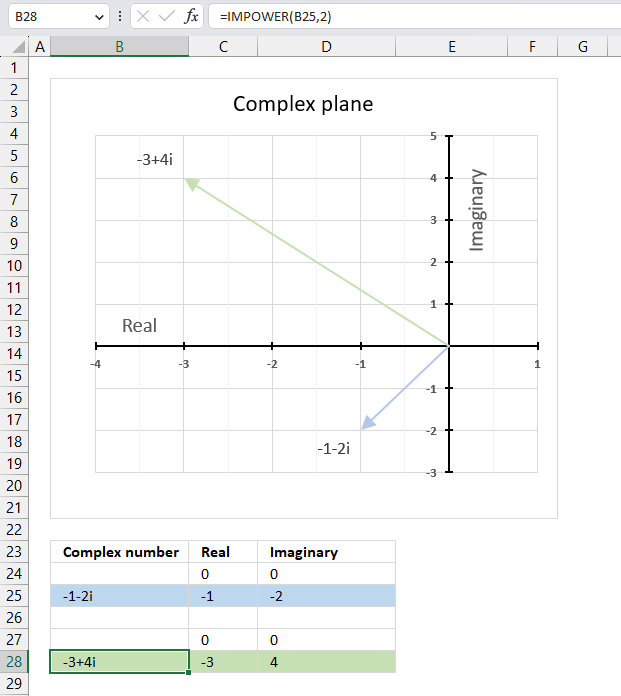The IMPOWER function calculates a complex number raised to a given power in x + yi or x + yj text format.

The letter j is used in electrical engineering to distinguish between the imaginary value and the electric current.

## 1. IMPOWER Function Syntax

IMPOWER(inumber, number)

## 2. IMPOWER Function Arguments

 inumber Required. A complex number in x+yi or x+yj text format. number Required. The power you want to raise the complex number to. Integer, fractional, or negative values are allowed.

## 3. IMPOWER function exampleThe image above demonstrates a formula in cell B28 that calculates a complex number specified in cell B25 raised to a power.

Formula in cell C3:

=IMPOWER(B25, 2)

The chart above shows the complex number -1-2i on the complex plane as a light blue line with an ending arrow, the green line with an ending arrow represents -1-2i raised to the power of 2 which is -2+4i.

### 3.1 Explaining formula

#### Step 1 - Populate arguments

IMPOWER(inumber, number)

becomes

IMPOWER(B3, 2)

#### Step 2 - Evaluate the IMPOWER function

IMPOWER(B3, 2)

becomes

IMPOWER("1+2i", 2)

and returns

-3+4i

## 4. Calculate a given complex number raised to a power in detailA complex number raised to a given power is calculated like this:

C = x + yi

Convert the complex number in rectangular form to polar form:
Z = r(cos θ + i sin θ)

θ = tan-1 (x/y)

r = √(x2+y2)

Raise the complex number in polar form to a power.

IMPOWER(C) = (x+y)n = rneinθ = rncos nθ+irnsin nθ

Convert the complex number in polar form to rectangular form.

x + yi = √(x2+y2)(cos θ + i sin θ)

### 4.1 Example: Raise -1-2i to the power of 2

C = -1-2i

θ = tan-1 (x/y) = tan-1 (-1/-2)  = tan-1 (0.5) = 0.463647609000806 radians. This is true if the complex number is in the first quadrant.

However, complex number -1-2i is in the third quadrant. We need to add π to 0.463647609000806 to get the correct radians.

π + 0.463647609000806 = 4.24874137138388

Example of quadrants in the complex plane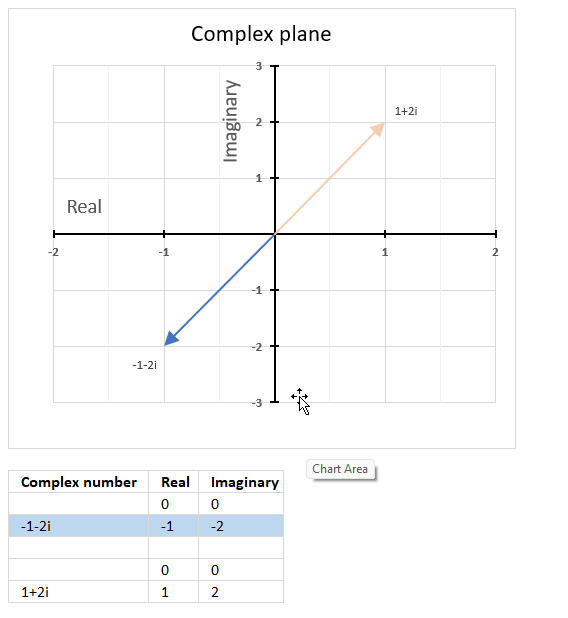The complex plane is divided into four quadrants, the orange line with an ending arrow represents 1+2i. It is in the first quadrant. The blue line with an ending arrow represents -1-2i, it is in the third quadrant.

r = √(x2+y2) = √((-1)2+(-2)2) = √(1+4) = √5

rncos nθ+irnsin nθ = (√5)2cos (2*4.24874137138388) + i(√5)2sin (2*4.24874137138388) = 5*cos (2*4.24874137138388) + i*5*sin (2*4.24874137138388) = 5*-0.6 + i*5*0.8 = -3+4i

You can also multiply x + yi by itself since we are raising it to the power of 2.

(x+yi)*(x+yi) = x2 + xyi + xyi -y2 = x2 + 2xyi - y2

i*i = -1

(-1-2i)*(-1-2i) = 1+2*2i-4 = -3+4i

## 5. What are quadrants in the complex plane?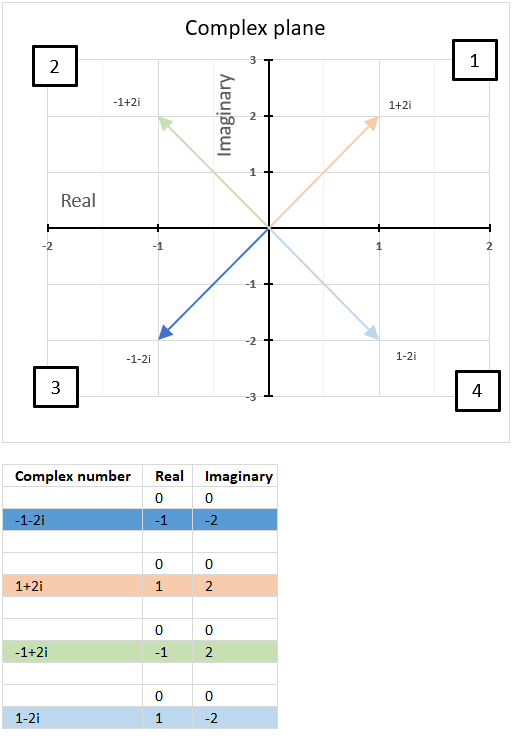Quadrants are four regions (Quad = 4) formed by the intersection of the real axis and the imaginary axis in the complex plane.

How are quadrants numbered in the complex plane?

They are numbered counterclockwise from 1 to 4 and starts from the region where both the real and imaginary parts are positive.

The chart above shows the complex plane with the corresponding quadrants and four complex numbers in each quadrant.

The first quadrant is defined as 0 < θ < π/2, the orange line represents 1+2i and is in the first quadrant.

The second quadrant is defined as π/2 < θ < π, the green line represents -1+2i and is in the second quadrant.

The third quadrant is defined as -π/2 < θ < -π, the blue line represents 1-2i and is in the third quadrant.

The fourth quadrant is defined as 0 < θ < -π/2, the light blue line represents -1-2i and is in the fourth quadrant.

Why is it important to know which quadrant a given complex number is located?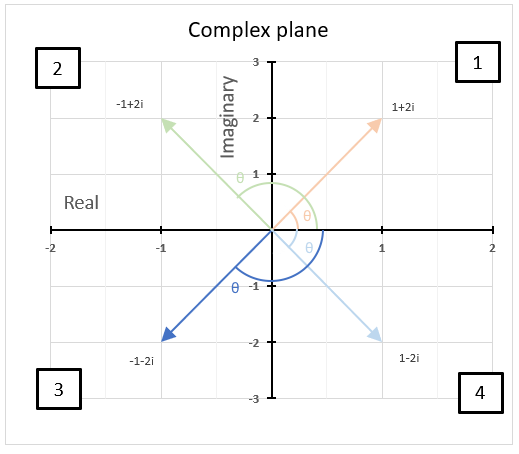It is important to know which quadrant a given complex number is in because it helps us determine the sign and angle. The angle of a complex number is between the line and the positive real axis.

The image above shows the angle or argument for the following four complex numbers:

## 6. Calculate the n-th root of a complex number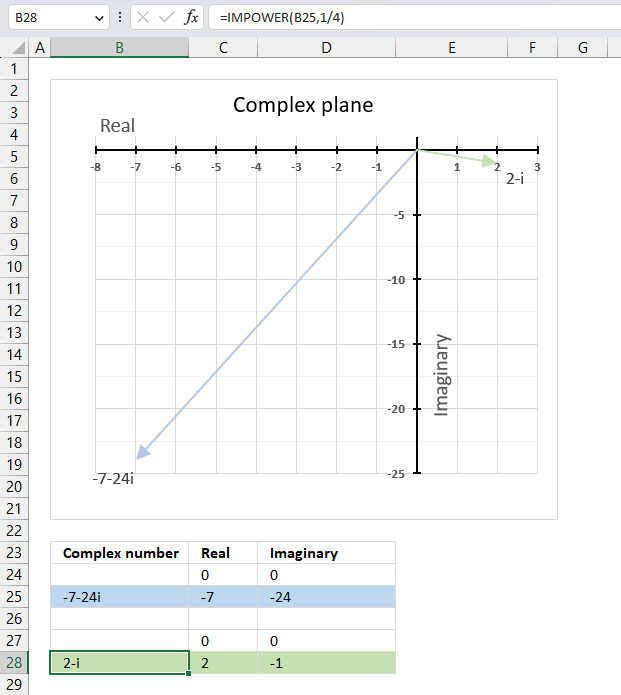The IMPOWER function lets you calculate the n-th root of a given complex number. To calculate the n-th root of a complex number divide 1 by n in the second argument. This is the same as complex_number^(1/n) which returns the n-th root.

IMPOWER(complex_number, 1/n)

The following formula calculates the 4-th root of complex number specified in cell B25.

Formula in cell B28:

=IMPOWER(B25,1/4)

### Explaining formula

1/n

becomes

1/4

#### Step 2 - Populate IMPOWER function

IMPOWER(inumber,number)

becomes

IMPOWER(B25,1/4)

#### Step 3 - Calculate the n-th root of a given complex number

IMPOWER(B25,1/4)

becomes

IMPOWER("-7,-24i",1/4)

and returns

"2-i"

### Get the Excel fileHow-to-use-the-IMPOWER-function.xlsx